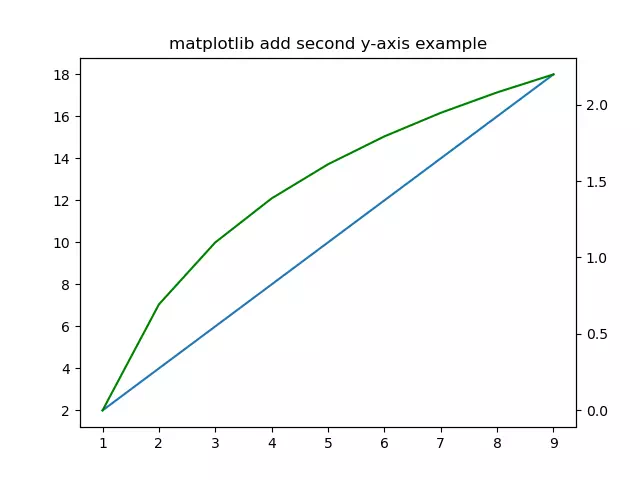# Matplotlib Second Y-Axis Label Example

This article will show you how to add the second y-axis with a label on Matplotlib plots.

### 1. How To Add The Second Y-Axis Label On Matplotlib Plot.

1. First plot the first curve with the default x, y-axis.
```# plot the first curve.
plt.plot(x,y1)```
2. Then call the matplotlib.pyplot module’s twinx() method to add the second y-axis on the figure right side.
```# add another y-axis.
plt.twinx()```
3. Now you can plot the second curve, and the second curve will use the same x-axis and the second y-axis.
```# plot the second curve, the curve line has green color.
plt.plot(x,y2,'g')```

### 2. Add The Second Y-Axis On Matplotlib Plot Example.

1. Below is the example full source code.
```import numpy as np
import matplotlib.pyplot as plt

def second_y_axis_example():

# create a numpy array contains integer number 1 - 10..
x=np.arange(1,10)
print('x: ', x)

# calculate y1.
y1 = x + x
print('y1: ', y1)

# calculate y2.
y2 = np.log(x)
print('y2: ', y2)

# plot the first curve.
plt.plot(x,y1)

# set the matplotlib plot title.

plt.twinx()

# plot the second curve, the curve line has green color.
plt.plot(x,y2,'g')

# display the plot.
plt.show()

if __name__ == '__main__':

second_y_axis_example()```
2. When you run the above example, you can get the below figure.This site uses Akismet to reduce spam. Learn how your comment data is processed.